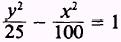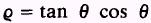Custom SearchADDITIONAL PRACTICE PROBLEMS 1. Find the equation of the curve that is the locus of all points equidistant from the point (-3,-4) and the line 6x - 8y = -2. 2. Find the coordinates of the center and the radius of a circle for the equation x2 + y2 - 10x = - 9. 3. Find the equation of the circle that passes through points ( - 4,3), (0, - 5), and (3, - 4). 4. Give the equation; the length of a; and the length of the focal chord for the parabola, which is the locus of all points equidistant from the point (0, - 23/4) and the line y = 23/4. 5. Reduce the equation 3x2 - 30x + 24y + 99 = 0 to a parabola in standard form. 6. Find the equation of the ellipse with its center at the origin, semimajor axis of length 14, and directrices y = 28. 7. Reduce the equation 4x2 + y2 - 16x - 16y = 64 to an ellipse in standard form. 8. Find the equation of the hyperbola with asymptotes at y = (4/3)x and vertices at ( 6,0). 9. Find the foci, directrices, eccentricity, equations of the asymptotes, and length of the focal chord of the hyperbola10. Change the equation x2 + 2x + y2 = 0 from rectangular to polar coordinates. 11. Change the equationto an equation in rectangular coordinates. ANSWERS TO ADDITIONAL PRACTICE PROBLEMSIntegrated Publishing, Inc. - A (SDVOSB) Service Disabled Veteran Owned Small Business